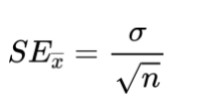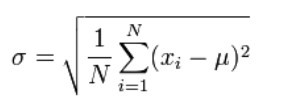# Standard Error Calculator

Enter the data in the below input box to find the standard error.The standard error calculator is used to find the dispersion of sample statistics. It provides you the options of entering raw data and summary data.

If the user opts for the raw data option, the standard error of the mean calculator gives the necessary data for the calculation of standard deviation i.e sum of the squares and mean.

## How to use the standard error calculator?

Follow the given instruction to use this tool:

1. Choose the type of calculator (raw or summary) according to the data you have.
2. Enter the numbers separated by commas for raw data.
3. For summary data, enter standard deviation and sample count.
4. Click calculate.

## What is the standard error?

The standard error tells us how far or close the mean of a sample is from the population mean. In other words, “the dispersion of the sample mean”.

The standard error is kind of a reliability check, to know how much the sample mean can be trusted. It is used in the fields of medicine, engineering, and many more.

## Standard error formula

The formula of standard deviation includes standard deviation as well. It is;Where the sigma symbol in the numerator represents standard deviation and the n in the denominator represents the sample.

## How to calculate the standard error?

The method of finding standard error depends on the data available. If one has raw data, one will first have to count the sample values, find the standard deviation, and use these values in the standard error formula.

On the other hand, if you already have the mentioned values, simply plug in the formula written above. You can double-check your answer from the standard error of the mean calculator.

Example:

What is the standard error of the following data?

1, 23, 4, 12, 10, 31

Solution:

Step 1: Count the values.

N = 6

Step 2: Find the standard deviation.

The formula is:Mean = (1, 23, 4, 12, 10, 31) / 6

= 81 / 6

= 13.5

Sum of the squares = (1-13.5)2 + (23-13.5)2 + (4-13.5)2 + (12-13.5)2 + (10-13.5)2 + (31-13.5)2

= 657.5

Putting the values in the formula

=(657.5 / 6)½

= 10.47

Step 3: Use this data in the standard error formula.

= 10.47/√6

= 4.274

Example 2:

Find the standard error if the variance of the sample data is 234 and the sample count is 7.

Solution:

Step 1: Write the given data.

Variance = 234

Count (N)= 7

Step 2: Find the standard deviation.

= Variance /N-1

= 234 / 7-1

= 234 / 6

= 39

Step 3: Use the standard error formula.

= 39 / √6

= 15.9